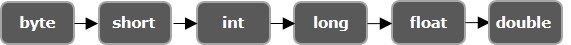# Explain widening with objects in Java.

Java provides various datatypes to store various data values. It provides 7 primitive datatypes (stores single values) namely, boolean, byte, char, short, int, long, float, double and, reference datatypes (arrays and objects).

Type Casting/type conversion − Converting one primitive datatype into another is known as type casting (type conversion) in Java. You can cast the primitive datatypes in two ways namely, Widening and, Narrowing.

Widening − Converting a lower datatype to a higher datatype is known as widening. In this case the casting/conversion is done automatically therefore, it is known as implicit type casting. In this case both datatypes should be compatible with each other.## Example

public class WideningExample {
public static void main(String args[]){
char ch = 'C';
int i = ch;
System.out.println(i);
}
}

## Output

Integer value of the given character: 67

## Widening with objects

you can cast the reference(object) of one (class) type to other. But, one of the two classes should inherit the other.

Therefore, if a class inherits the properties of the other conversion of sub class object in to super class type is considered as widening with respect to objects.

## Example

In the following Java example, we have two classes namely Person and Student. The Person class has two instance variables name and age and one instance method displayPerson() which displays the name and age.

The Student extends the person class and in addition to the inherited name and age it has two more variables branch and student_id. It has a method displayData() which displays all four values.

In the main method, objects of the both classes created separately and, the super class object is converted in to sub class object.

class Person{
private String name;
private int age;
public Person(String name, int age){
this.name = name;
this.age = age;
}
public void displayPerson() {
System.out.println("Data of the Person class: ");
System.out.println("Name: "+this.name);
System.out.println("Age: "+this.age);
}
}
public class Student extends Person {
public String branch;
public int Student_id;
public Student(String name, int age, String branch, int Student_id){
super(name, age);
this.branch = branch;
this.Student_id = Student_id;
}
public void displayStudent() {
System.out.println("Data of the Student class: ");
System.out.println("Name: "+this.name);
System.out.println("Age: "+this.age);
System.out.println("Branch: "+this.branch);
System.out.println("Student ID: "+this.Student_id);
}
public static void main(String[] args) {
//Creating an object of the Student class
Student student = new Student("Krishna", 20, "IT", 1256);
//Converting the object of Student to Person
Person person = new Person("Krishna", 20);
//Converting the object of student to person
person = (Student) student;
person.displayPerson();
}
}

## Output

Data of the Person class:
Name: Krishna
Age: 20

In short, super class reference variable can hold the sub class object. But, using this reference you can access the members of super class only, if you try to access the sub class members a compile time error will be generated.

public static void main(String[] args) {
Person person = new Student("Krishna", 20, "IT", 1256);
person.displayStudent();
}

## Output

Student.java:33: error: cannot find symbol
person.dispalyStudent();
^
symbol: method dispalyStudent()
location: variable person of type Person
1 error